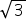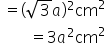Maths-
General
Easy

Question

# A square is drawn with the length of the side equal to the diagonal of the cube. if the square area is 72075 cm2, then find the side of the cube?Hint:

## The correct answer is: 155 cm.

### The length of the square and the diagonal of the cube are coincided. Therefore, the value of the two will be equal. We are given that, Area of the square=72,075 cm²Side of square=Diagonal of cubeLet the edge of the cube be 'a' cm.As we know,length of the diagonal of cube=×edge of the cube=a cm The length of the side of the square is equal to the length of the diagonal of the cube. Area of square(drawn on diagonal of cube)We know that, area of square drawn on the diagonal of a cube= 72,075 cm² Therefore,Therefore , the side of the cube is 155 cm.#### With Turito Foundation.#### Get an Expert Advice From Turito.